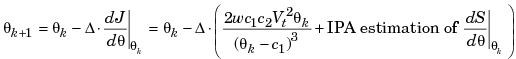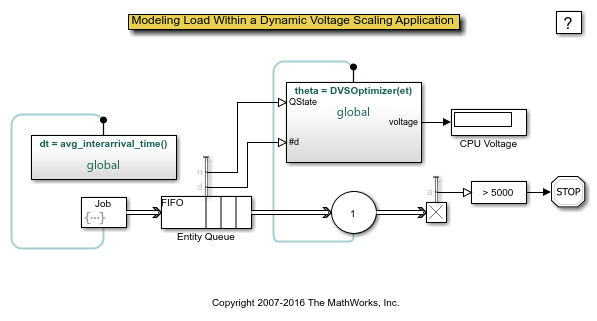Main Content

# Modeling Load Within a Dynamic Voltage Scaling Application

### Overview

This example shows how, depending on the workload, a AT90S8535 microcontroller uses a dynamic voltage scaling (DVS) feature to adjust the input voltage. By lowering the input voltage when the workload is low, the microcontroller reduces energy consumption while guaranteeing quality of service. The DVS controller is based on an online gradient estimation technique called infinitesimal perturbation analysis (IPA). In a single simulation of a parameterized system, not the large number of simulations required by a traditional finite-difference approach, IPA can provide sensitivity information that yields a first-order approximation of the system performance metrics as a function of the parameters.

### Applying IPA to the Controller

The performance metric to minimize is the average cost per job, given bywhere

•is the average service time of a job, which is a function of the input voltage V. That is, finding the optimal value ofalso yields the optimal value of V.

•is a weighting constant.

•is the average energy consumption of a job in Joules.

•is the average system time for jobs, which measures quality of service. This model uses an M/M/1 queuing system, so a closed-form expression forprovides a way to compare the IPA results in the simulation with theoretical results.

•andare device-dependent constants.

•is the device minimum input voltage.

To find a value offor whichis 0, this model uses a gradient method with constant step size. Theth iteration of the optimization, which occurs upon the departure of theth job, uses the estimateto produceTo learn about the IPA estimation of, see the works listed in References.

### Structure of the Model

The model includes these components:

• Job Arrivals section: Provides source of jobs that form the workload

• FIFO Queue, Single Server, and other blocks in the blue section: Provides queuing for jobs in the system

• DVS Optimizer subsystem: Uses the queue length,value, service time for the latest job, and total number of jobs to computeand the corresponding updated input voltage.### Results and Displays

The model includes these visual ways to understand its performance:

• A dynamic plot showing how the DVS controller varies the voltage during the simulation to reduce the average cost per job.

• A Display block that shows the average service time for jobs.

• A Display block that shows the corresponding input voltage.

To experiment, try changing the value of the Avg Interarrival Time block before running the simulation.### References

 Cassandras, C. G., and S. Lafortune. Introduction to Discrete Event Systems. Boston, MA: Kluwer Academic Publishers, 1999.

 Li, W., C. G. Cassandras, and M. I. Clune. "Model-Based Design of a Dynamic Voltage Scaling Controller Based on Online Gradient Estimation Using SimEvents." Proceedings of 45th IEEE Conference on Decision and Control. 2006, pp. 6088-6092.

 Weiser, M., B. Welch, A. Demers, and S. Shenker. "Scheduling for Reduced CPU Energy." Proceedings of the 1st Symposium on Operating Systems Design and Implementation. 1994, pp. 13-23.

## SupportGet trial now